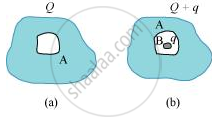HSC Science (General) 11thMaharashtra State Board
Share

# A Conductor a with a Cavity as Shown in Fig. 1.36(A) is Given a Charge Q. Show that the Entire Charge Must Appear on the Outer Surface of the Conductor. - HSC Science (General) 11th - Physics

ConceptContinuous Charge Distribution

#### Question

(a) A conductor A with a cavity as shown in Fig. 1.36(a) is given a charge Q. Show that the entire charge must appear on the outer surface of the conductor. (b) Another conductor B with charge is inserted into the cavity keeping B insulated from A. Show that the total charge on the outside surface of A is [Fig. 1.36(b)]. (c) A sensitive instrument is to be shielded from the strong electrostatic fields in its environment. Suggest a possible way.#### Solution

Let us consider a Gaussian surface that is lying wholly within a conductor and enclosing the cavity. The electric field intensity E inside the charged conductor is zero.

Let q is the charge inside the conductor and in_0is the permittivity of free space.

According to Gauss’s law,

Flux, phi=vecE.vec(ds)=q/in_0

Here, = 0

q/in_0=0

in_0ne0

therefore q=0

Therefore, charge inside the conductor is zero.

The entire charge Q appears on the outer surface of the conductor.

(b) The outer surface of conductor A has a charge of amount Q. Another conductor B having charge +qis kept inside conductor A and it is insulated from A. Hence, a charge of amount −will be induced in the inner surface of conductor A and +q is induced on the outer surface of conductor A. Therefore, total charge on the outer surface of conductor A is Q q.

(c) A sensitive instrument can be shielded from the strong electrostatic field in its environment by enclosing it fully inside a metallic surface. A closed metallic body acts as an electrostatic shield.

Is there an error in this question or solution?

#### APPEARS IN

NCERT Solution for Physics Textbook for Class 12 (2018 to Current)
Chapter 1: Electric Charge and Fields
Q: 28 | Page no. 49

#### Video TutorialsVIEW ALL 

Solution A Conductor a with a Cavity as Shown in Fig. 1.36(A) is Given a Charge Q. Show that the Entire Charge Must Appear on the Outer Surface of the Conductor. Concept: Continuous Charge Distribution.
S